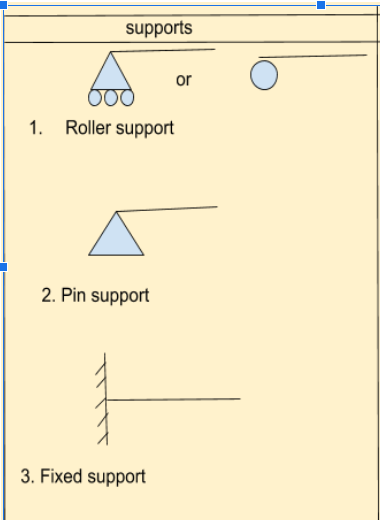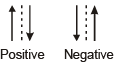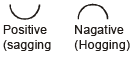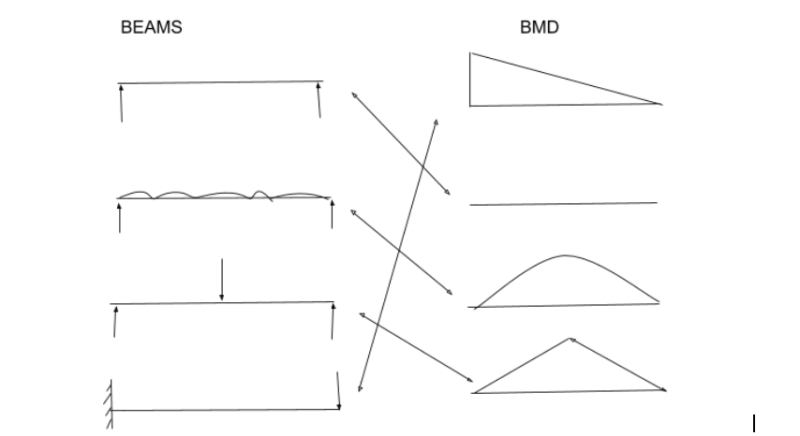Courses

# Shear force and bending moment diagram. Civil Engineering (CE) Notes | EduRev

## Civil Engineering (CE) : Shear force and bending moment diagram. Civil Engineering (CE) Notes | EduRev

The document Shear force and bending moment diagram. Civil Engineering (CE) Notes | EduRev is a part of Civil Engineering (CE) category.
All you need of Civil Engineering (CE) at this link: Civil Engineering (CE)

created by - premprakash

LESSON

## DEFINITION OF BEAM

• beam a structure member that has a long length as compare to breath or dia.
• it subjected to transverse loads, couple.

TYPES OF SUPPORT

1. roller support
2. pin support
3. fix support

## 1. roller support

• allows rotation.
• allows translation.
• have 1 vertical reaction.

## 2. pin support or hinge support.

• allows rotation
• have 2 reaction- one vertical, one horizontal

## 3. fix support

• no rotation
• no translation
• have 3 reactions- one vertical, one moment, one horizontal reaction.## 3. fixed beam

• both ends of the beam are fixed.

## 4. continuous beam.

• rest on more than two support throughout its length.

## 5. overhang beam.

• extends from its support either one side or both sides.
• it has features of simply supported and cantilever beam.

a beam may be subjected to either or in combination of the following loads.

1. concentrated or point load- it is load acting on a single point.
2. uniformly distributed load(UDL)- it is load uniformly spread over the beam and in calculation assumed acts at c.g. point throughout its length.
3. uniformly varying load(UVL)- it is load varying at one end to the other end and sometimes is known as a triangular load.

## 1. SHEAR FORCE

• algebraic sum of all transverse forces either to the left or right hand of the section.
• it is written as a symbol as S.F. or F only.

## 2. bending moment

• algebraic sum of moments of all transverse force either to the left or right of the section.
• it is written as symbol B.M. OR M only.

• ## calculation of shear force at section or point, generally we take all the sum of forces either left or right of the section that is SF at that section.

• if assume we take the sum of all forces right of the section then upward forces are taken negative and downward forces are taken as positive in calculation of shear force.## 2. for bending moment calculation• in calculation of bending moment, we take the sum of all the moment either right or left of at section or point that is bending moment at that section.
• assume we take the right side of forces in calculation of bending moment, if that force cause sagging of the beam then called positive bending moment.

• ## draw of bending moment throughout, length of the beam is called bending moment diagram(BMD)

 types of loading variation of shear force variation of moment point rectangle linear uniformly distributed linear square parabola uniformly varying parabolic cubic parabola parabolic cubic fourth-degree polynomial couple or moment no shear variation a vertical step at point of application

## RELATION BETWEEN LOAD, S.F., B.M.

• rate of change of shear force is equal to load.
• dF/dx = W
• rate of change of bending moment is equal to shear force
• dM/dx = F
• bending moment is maximum when the shear force is zero or change sign.
• point of contra flexure where bending moment is zero or change sign.

MULTIPLE CHOICE QUESTIONS PART 1

1. if a cantilever beam is subjected to point load at its free end then shear force under the load is - equal to the load.
2. the bending moment at the free end of a cantilever beam carrying any type of load is - zero
3. the bending moment at the center of a simply supported beam carrying a uniformly distributed load is - wl2/8
4. when the shear force at that point is zero, the bending moment at that point will be - max
5. the point of contra flexure is a point where - bending moment change sign
6. the shear force in a beam subjected to pure positive bending is - zero
7. the number of independent reaction components for a hinge or pinned support is - 2
8. the shape of the shear force diagram for a cantilever subjected to a couple at its free end is - no shear force in any part of the beam.
9. the bending moment in a beam is maximum where - shear force change sign or zero.
10. match the list 1 and list 2thankyou created by premprakash

Offer running on EduRev: Apply code STAYHOME200 to get INR 200 off on our premium plan EduRev Infinity!

,

,

,

,

,

,

,

,

,

,

,

,

,

,

,

,

,

,

,

,

,

;